# FPROPS/Thermal conductivity

Calculation of thermal conductivity in FPROPS is in development. This development is driven by an application requiring transport properties of carbon dioxide, so the first correlations being implemented will be for that. Although textbooks such as Incropera and DeWitt, Holman and Cengel use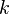$k$ for thermal conductivity, most publications on thermophysical properties use the convention of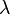$\lambda$, and that convention will be used on this page.

Correlations typically specify conductivity in terms of ideal (zero-density limit) part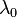$\lambda_0$, residual part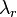$\lambda_r$ and critical region enhancement function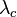$\lambda_c$, as follows: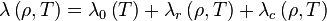$\lambda \left(\rho,T \right) = \lambda_0 \left(T \right) + \lambda_r \left(\rho, T\right) + \lambda_c \left(\rho, T\right)$

## Ideal part

The ideal (zero-density limit) part has been expressed in several places as: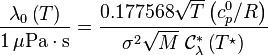$\frac{\lambda_0\left(T\right)}{1\,\mathrm{\mu Pa\cdot s}} = \frac{0.177568 \sqrt{T} \left(c_p^0/R\right)}{\sigma^2 \sqrt{M} \; \mathcal{C}_\lambda^\ast\left(T^\star\right)}$.

Here,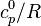$c_p^0/R$ is the isobaric heat capacity divided by the gas constant (either both specific or both molar),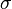$\sigma$ is length scaling parameter (in units of nm, which is messy), a constant particular to the fluid in question, and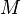$M$ is relative molecular mass of the fluid.

The reduced temperature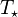$T_\star$ is calculated as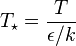$T_\star = \frac{T}{\epsilon / k}$
where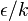$\epsilon / k$ is the 'energy scaling parameter', specific to the fluid in question. The reduced effective cross-section for thermal conductivity,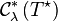$\mathcal{C}_\lambda^\ast \left(T^\star\right)$ (written with the mysterious
Error creating thumbnail: File missing
symbol in several publications) is usually provided in the form of a power series like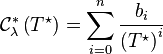$\mathcal{C}_\lambda^\ast \left(T^\star\right) = \sum_{i=0}^{n}{\frac{b_i}{\left(T^\star\right)^i}}$

where the constants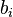$b_i$ would be tabulated for each fluid separately.

Essentially, the ideal part of the thermal conductivity, then, is a ratio of two power series in terms of$T$: one derived from experimentally-determined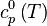$c_p^0\left(T\right)$, and the other a reduced effective cross-section derived experimentally from low-density conductivity data,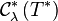$\mathcal{C}_\lambda^\ast \left(T^\ast\right)$. To accommodate the many different ways that authors may publish their results, we can allow arbitrary power series on both numerator and denominator, and we can allow the already-defined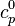$c_p^0$ function to be referenced/used as the denominator if desired.

The calculation of the effective cross section$\mathcal{C}_\lambda^\ast \left(T^\ast\right)$ can be generalised a little better by defined it as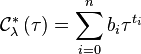$\mathcal{C}_\lambda^\ast \left(\tau\right) = \sum_{i=0}^{n} b_i \tau^{t_i}$

with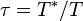$\tau = T^\ast / T$, and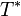$T^\ast$ is a scaling temperature provided as part of the correlation data. The specific correlation above would be writen with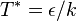$T^\ast = \epsilon / k$ hence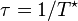$\tau = 1 / T^\star$.

(Note that the reduced effective cross section is dimensionless, and is reduced from the 'real' effective cross-section area using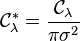$\mathcal{C}_\lambda^\ast = \frac{\mathcal{C}_\lambda}{\pi \sigma^2}$.)

Note that one publication provides the function$\lambda_0$ in the form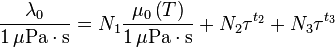$\frac{\lambda_0}{1\,\mathrm{\mu Pa \cdot s}} = N_1 \frac{\mu_0\left(T\right)}{1\,\mathrm{\mu Pa \cdot s}} + N_2 \tau^{t_2} + N_3 \tau^{t_3}$

and this form does not comply with the above ratio of power series, since zero-density viscosity is, in turn, defined as a ratio of power series (see FPROPS/Viscosity). This form could be accommodated by 'flagging' one of the terms in the numerator power series in a special way, and providing a pointer to the appropriate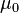$\mu_0$ data/function, perhaps.

## Residual part

The residual part is correlated by Lemmon and Jacobsen as: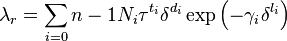$\lambda_r = \sum_{i=0}{n-1} N_i \tau^{t_i} \delta^{d_i} \exp\left(-\gamma_i \delta^{l_i}\right)$

with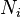$N_i$,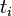$t_i$,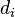$d_i$,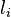$l_i$ all given as correlation data for the particular fluid, and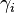$\gamma_i$ defined as zero when$l_i$ is zero, or one otherwise.

Lemmon and Jacobsen define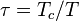$\tau = T_c / T$ and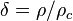$\delta = \rho / \rho_c$; but for flexibility, we can define arbitrarily-scaled temperature and density, with$T^\ast$ and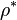$\rho^\ast$ provided as part of the correlation data,$\tau = T^\ast / T$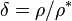$\delta = \rho / \rho^\ast$

Vesovic et al find that they can represent the residual part for carbon dioxide using power series in terms only of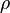$\rho$, as follows: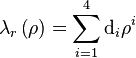$\lambda_r \left(\rho \right) = \sum_{i=1}^{4} \mathrm{d}_i \rho^i$.

This form can be accommodated by the form of Lemmon and Jacobsen by setting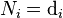$N_i = \mathrm{d}_i$,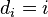$d_i = i$, and$t_i$ and$l_i$ to zero, and$\rho^\ast$ and$T^\ast$ to one.

## Critical part

The approach attributed to Olchowy and Sengers is used in both the carbon dioxide data and nitrogen data, although the equations presented in each of these references appear somewhat different (Specifically, in Lemmon and Jacobsen,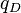$q_D$ has replaced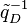${\tilde q}_D^{-1}$ from Vesovic et al, and the function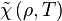$\tilde\chi \left(\rho,T\right)$ has been divided by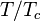$T/T_c$). Using the formulae of Lemmon and Jacobsen, the following parameters are required as correlation data:

symbol value and units (for CO2) name$q_D$ 4.0e-10 m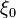$\xi_0$ 1.5e-10 m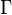$\Gamma$ 0.052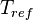$T_{ref}$ 450 K

There are also several 'universal' theoretical constants in the Olchowy and Sengers equations: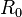$R_0$= 1.01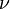$\nu$= 0.630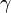$\gamma$= 1.2415

We define the 'reduced symmetrized compressibility' as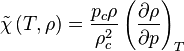$\tilde{\chi}\left(T,\rho\right) = \frac{p_c \rho}{\rho_c^2} \left(\frac{\partial \rho}{\partial p}\right)_T$

then the critical enhancement to thermal conductivity is calculate from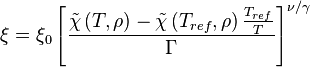$\xi = \xi_0 \left[ \frac{\tilde\chi\left( T,\rho \right) - \tilde\chi \left( T_{ref},\rho \right) \frac{T_{ref}}{T}}{\Gamma} \right]^{\nu/\gamma}$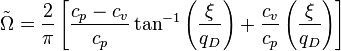$\tilde\Omega = \frac{2}{\pi} \left[ \frac{c_p - c_v}{c_p} \tan^{-1} \left(\frac{\xi}{q_D}\right) + \frac{c_v}{c_p} \left(\frac{\xi}{q_D}\right) \right]$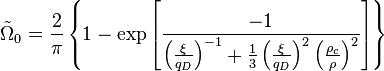${\tilde\Omega}_0 = \frac{2}{\pi} \left\{ 1 - \exp \left[ \frac{-1}{ \left(\frac{\xi}{q_D}\right)^{-1} + \frac{1}{3} \left(\frac{\xi}{q_D}\right)^2 \left(\frac{\rho_c}{\rho}\right)^2} \right] \right\}$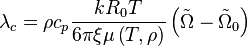$\lambda_c = \rho c_p \frac{k R_0 T}{6 \pi \xi \mu\left(T,\rho\right)} \left(\tilde\Omega - {\tilde\Omega}_0 \right)$